# Numbers in Excel

### How are numbers stored, formatted and calculated?

When dealing with numbers in Excel we should be aware of a few things:

• numbers in Excel can be formatted in many ways: plain numbers, currency, percentage, date etc.
• no matter how they are formatted and possibly edited, underlying values saved in cells are still decimal numbers
• those decimal numbers that Excel uses and calculates with go up to 15 decimal places (and even with that, there are caveats)
• this is not changed even if you force Excel to show more decimal places.

Let’s look at this example: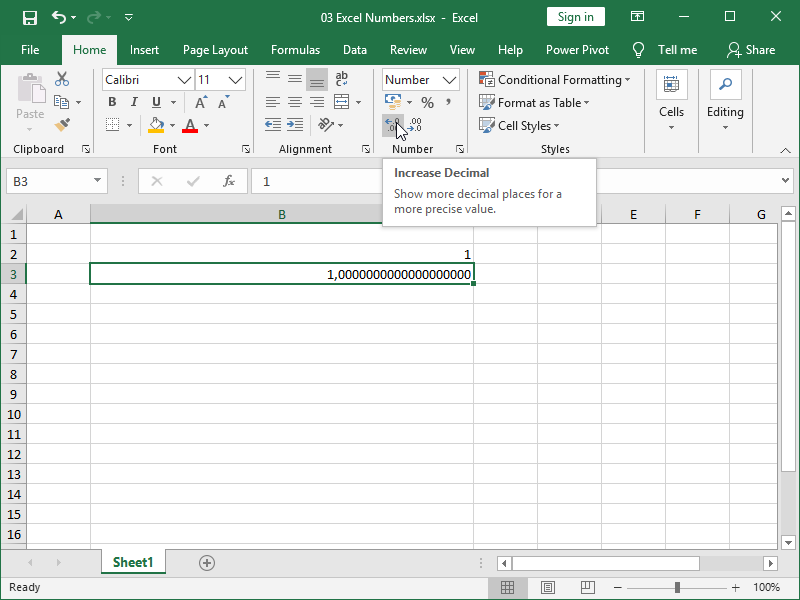Cells B2 and B3 contain the same number – only difference is that we changed the number of decimals shown with Increase Decimal button. Decrease Decimal button to the right of it works the other way around. Again, the same value, this is only formatting.

Let’s look at the next example: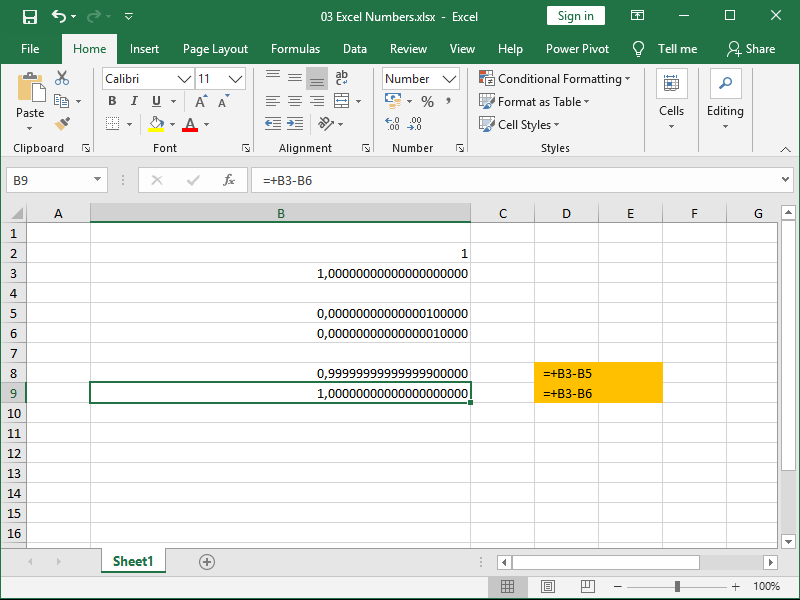As you can see, Excel will ignore the 16th decimal when calculating difference between B3 and B6 cells.

This limited accuracy is done for the sake of calculation speed and efficiency, and is more than good enough for most purposes. Nevertheless, keep this in mind if and when you start building really complex tables.

Let’s now look at the different ways we can format number 0,5 if we right-click on a cell and select Format Cells: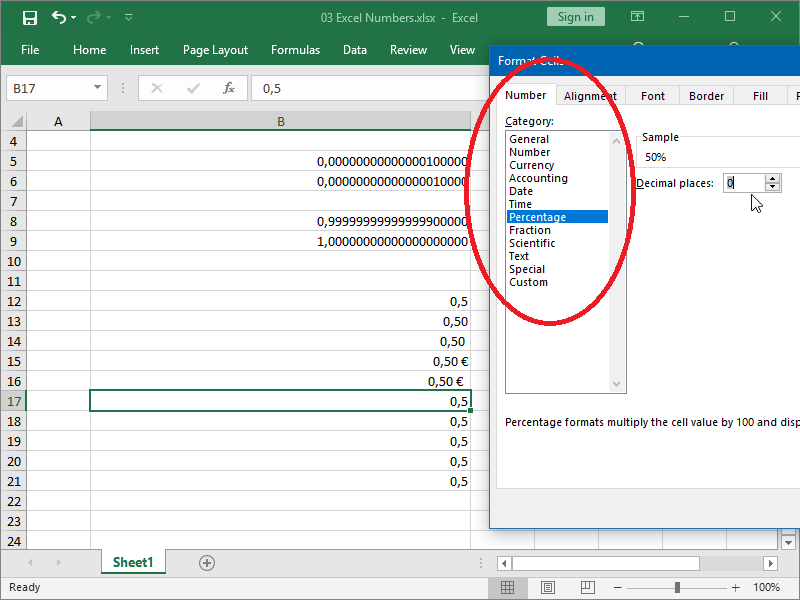You can see that almost all of the formatting options available are for numbers. Only Text and some Custom formats are not intended for numbers.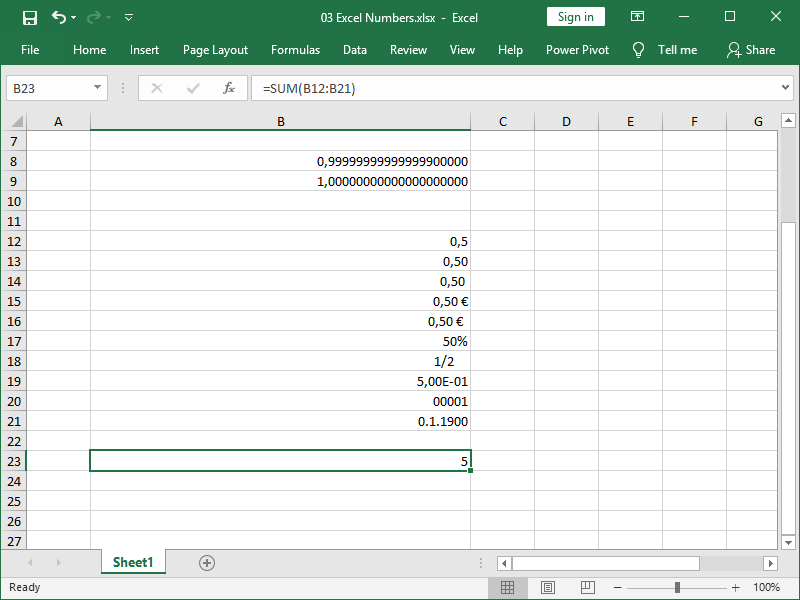Note again how, no matter the format, our formulas can still perform calculations on numbers in cells.

### How to convert text to number?

Currency amounts, percentages, fractions and dates are all intended to be numbers. However, that is not always the case, and text formatting combined with manual editing of text fields is common.

Still, those numbers need to be numbers, however formatted, if we wish to use them in calculations and avoid manual data processing. If we receive such data, we will then have to convert it back to numbers.

In our next example we have a single data export with several columns of numbers formatted as text. Column stars show user ranking for mobile game, column level their in-game progress, and column charge amount users should be charged. Notice how cells in columns C and D have error warnings in the form of green triangles in the upper left corner of each cell: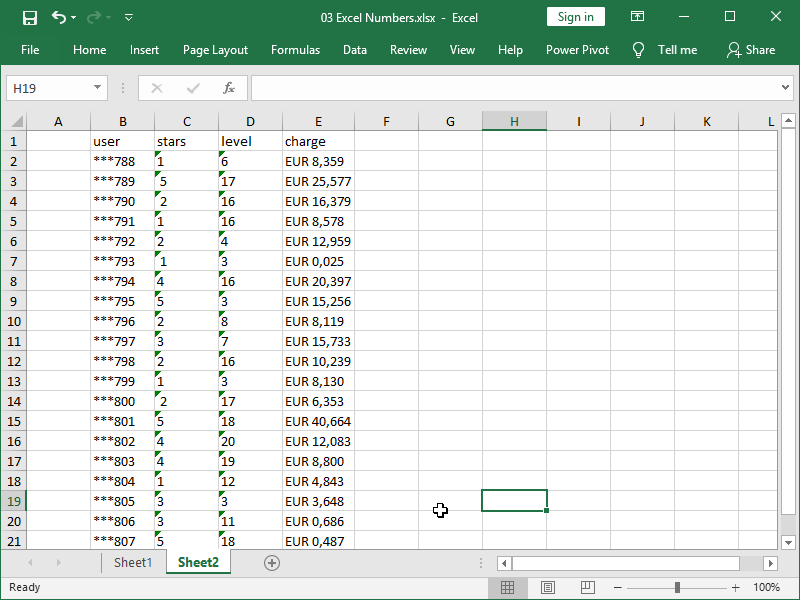If we select one or more of those cells exclamation mark button will appear, and if we left-click on in, a menu. Numbers in this column are not perfectly formatted (there are some spaces around numbers), but this built in feature Convert to Number will nevertheless be able to convert those numbers saved as text to numbers: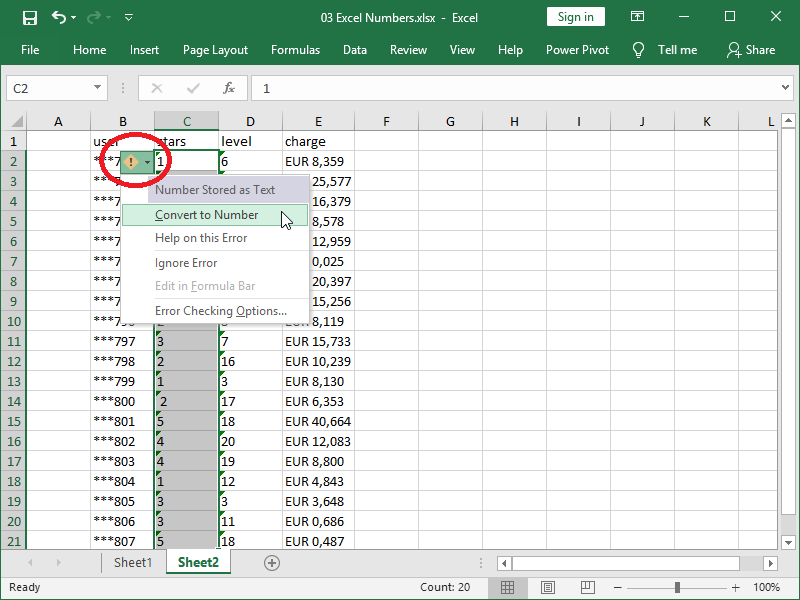If we however don’t want to override existing data, we can use a function for the same task.

This can be easiest done with the VALUE function, which converts a text string that represents a number to a number.

We will now convert values in column D to numbers: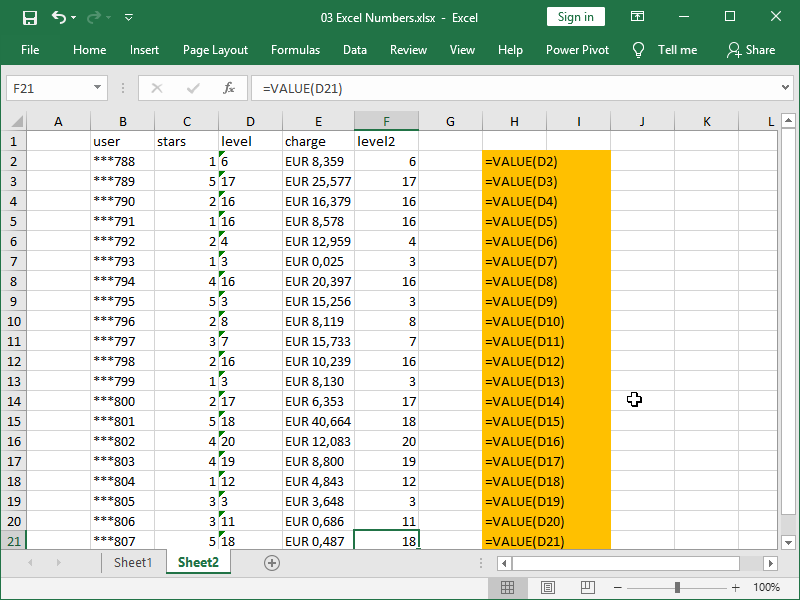Numbers in this column are also not perfectly formatted (there are again some spaces around numbers), but the VALUE function will also be able to deal with that.

What will however have an issue with the column E. If text there would be formatted as, for example, € 8,359, our job would be a lot easier. As it is, Excel can’t recognize that EUR is a currency symbol, so we will have to trim that part or the text before conversion: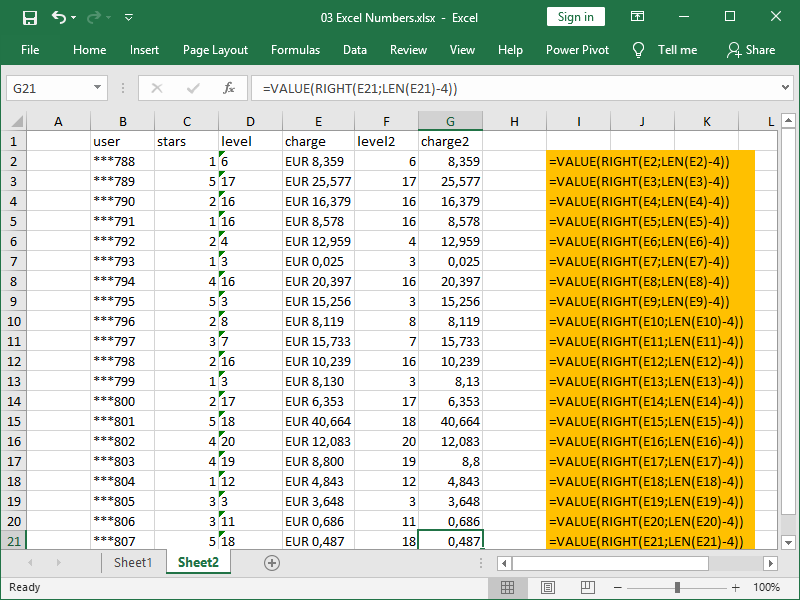As you can see, we are returning (length of the text string minus 4) right number of characters into the VALUE function, which than converts them to number.

If needed, now we can format our cells to currency format via right-click Format Cells > Currency: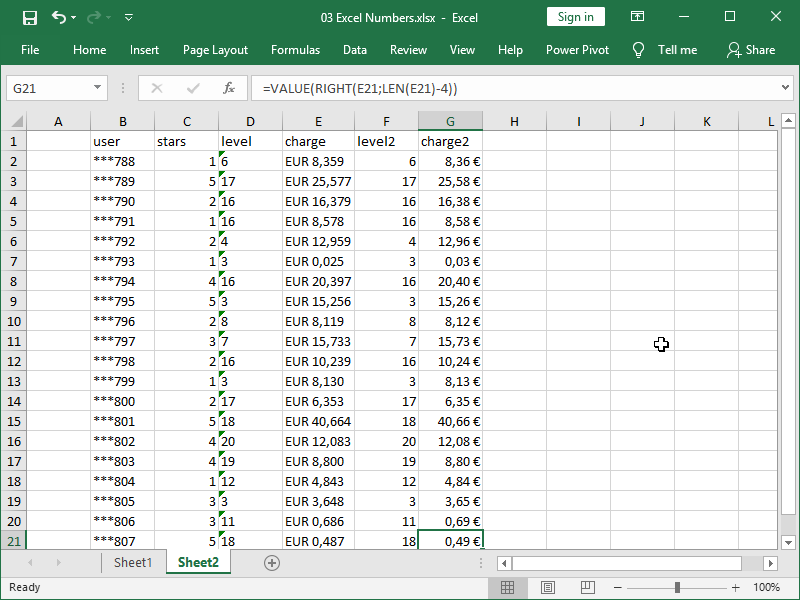### Basic math functions

Here are some already shown and some new math functions:

The SUM function returns added values.

The COUNT function returns the number of cells that contain numbers.

The ABS function returns the absolute value of a number – for example, =ABS(-7) or =ABS(7) returns 7.

The RAND function returns random number between 0 and 1, and RANDBETWEEN function returns random whole number between two numbers specified.

The POWER function returns the result of a number raised to a power – for example, =POWER(5;2) returns 25.

The SQRT function returns a positive square root – for example, =SQRT(25) returns 5.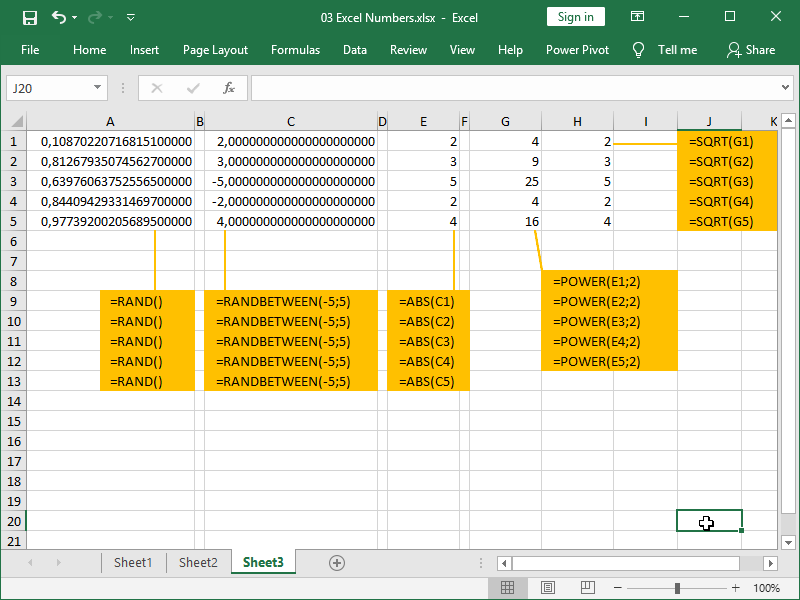As briefly mentioned in the introduction part, we can also use exponentiation operator [^] to perform exponentiation:

=5^2 returns 25, while =25^(1/2) returns 5

=5^3 returns 125, while =125^(1/3) returns 5, etc.

In a way, POWER and SQRT functions in Excel are not exactly needed – you will often encounter more than one way to do certain tasks in Excel.

Another common thing to do with numbers in Excel is to round them using a function.

In our previous example, we’ve already shown charge2 column that contains decimal numbers rounded to up to three decimals, and is formatted in a way where it’s always showing us two decimals.

That may be fine if we want to just look at those numbers, but we naturally can’t charge those amounts rounded to three decimals to customers, and we need to have the figures we are actually charging them.

If we want to limit our rounding to some other number of decimals, we can use the ROUND function.

Note that the ROUND function is not a formatting function, it will not just hide the third decimal like Decrease Decimal button, or formatting our number as currency, would. Here, we will create a new number, i.e. instead of a number 8,359 we will have a number 8,36.

Let’s take a look: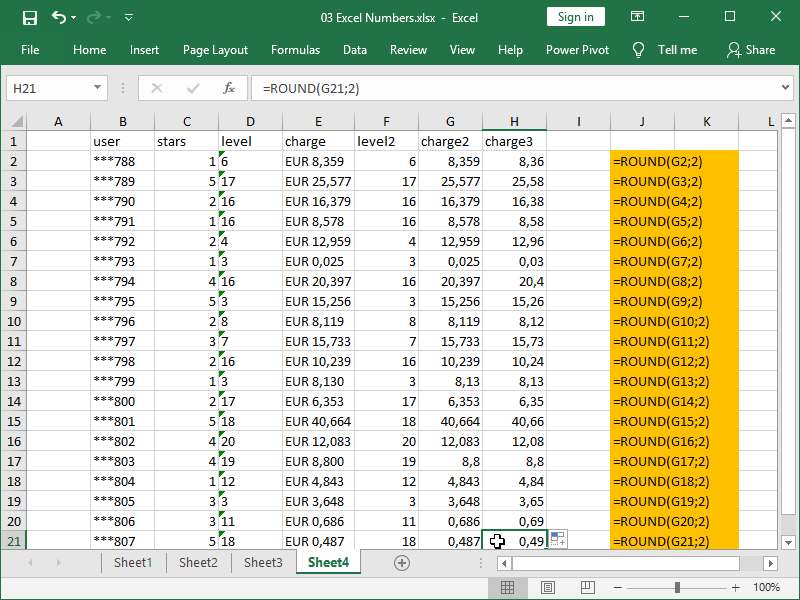As you can see, first we are referring to a cell, and next defining number of digits rounding to round to.

ROUNDUP and ROUNDDOWN work the same way, but instead of proper rounding they are rounding every number either up or down. Here is how that works: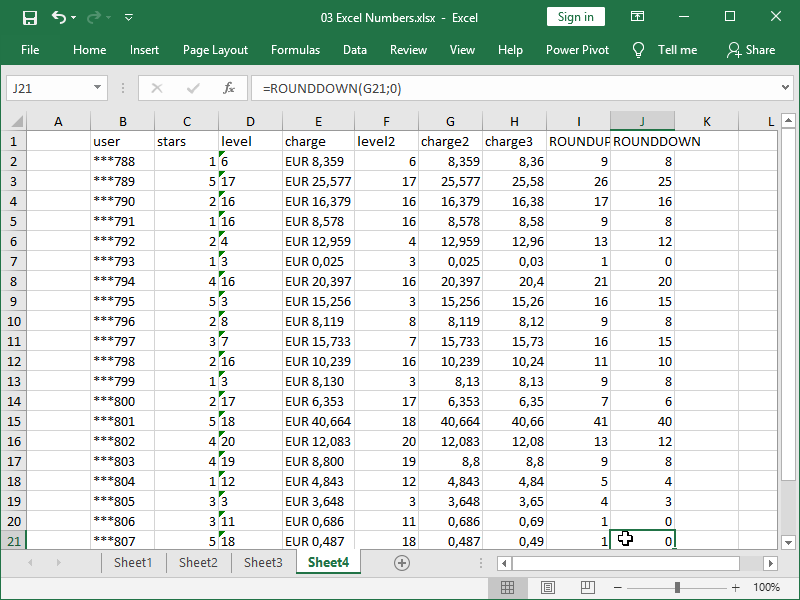ROUNDUP always rounds up away from zero, i.e. -1,7 would be rounded to -2, while ROUNDDOWN always rounds towards zero, i.e. -1,7 would be rounded to -1.

### Basic statistical functions

The MIN function returns the smallest number.

The MAX function returns the largest number.

The AVERAGE function returns the arithmetic mean of the arguments.

The MODE.SNGL function returns the most common number. If all of the numbers are non-repeating, error will be returned as there is no mode. If there are multiple modes, the first on the list, as is sorted, will be returned.

The MODE function is an older version of the MODE.SNGL function and behaves in the same way, while the MODE. MULT function can return multiple modes.

This is demonstrated in our next example, while both numbers 1 and 2 occur five times, MODE.SNGL function returns number 1 as mode: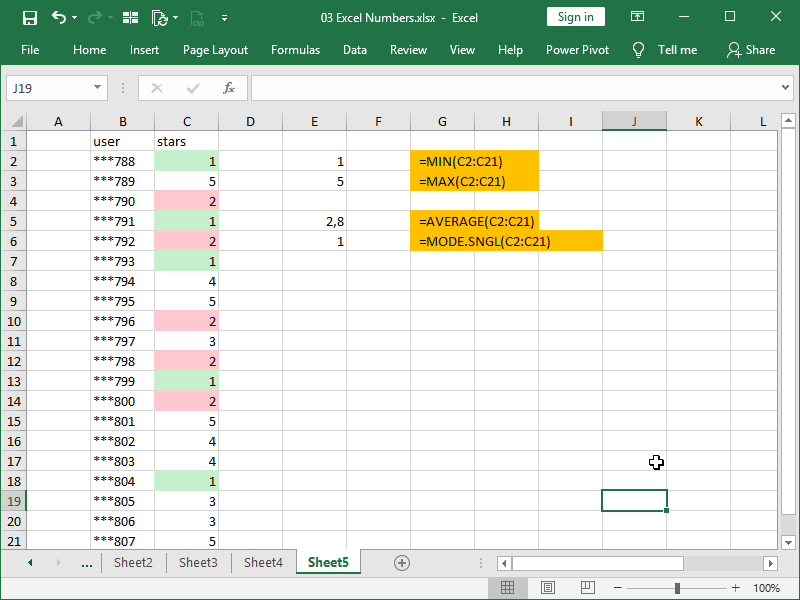Quantiles are defined as cut points dividing the list of numbers into the equal sized groups or intervals, with one fewer quantile than the number of groups created. Some of them have special names, such as median, quartiles, deciles, and percentiles. The groups created are called halves, quarters, etc.

There are multiple methods of quantile calculation and Excel is using linear interpolation between adjacent ranks, where rank x is equal to (N+1-2C)*p+C, with two variants:

• .INC formulas variant of the calculation includes both endpoints of the range (0,1), while assuming that C=1, x=(N-1)*p+1
• .EXC formulas variant of the calculation excludes both endpoints of the range (0,1) while assuming that C=0, x=(N+1)*p.

C represents constant, x represents rank (location of the percentile in the list of numbers), p represents target quantile and N represents number of observations.

Both variants are valid, with the first one historically being more commonly used in Excel, and the second one in various dedicated statistical applications.

Excel is not exactly consistent with functions calculating quantiles, with percentile functions serving as general purpose quantile functions.

The PERCENTILE.INC function returns the k-th quantile of values in a range, where k is in the range from 0 to 1, inclusive.

The PERCENTILE function is an older version of the PERCENTILE.INC function and behaves in the same way.

The PERCENTILE.EXC function returns the k-th quantile of values in a range, where k is in the range from 0 to 1, exclusive.

Note the naming scheme here, Excel function such as PERCENTILE functions are using “k-th” instead of “n-th”, and you will see both used interchangeably in various tutorials.

The MEDIAN function returns the median, the number in the middle of a set of numbers, with half of the numbers under the median, and half over the median.

The QUARTILE.INC function returns the of 1st, 2nd or 3rd quartile, or maximum value of values in range, inclusive.

The QUARTILE function is an older version of the QUARTILE.INC function and behaves in the same way.

The QUARTILE.EXC function returns the returns the of 1st, 2nd or 3rd quartile, exclusive.

Returns made by these functions can also be returned by PERCENTILE functions, as demonstrated in the following example: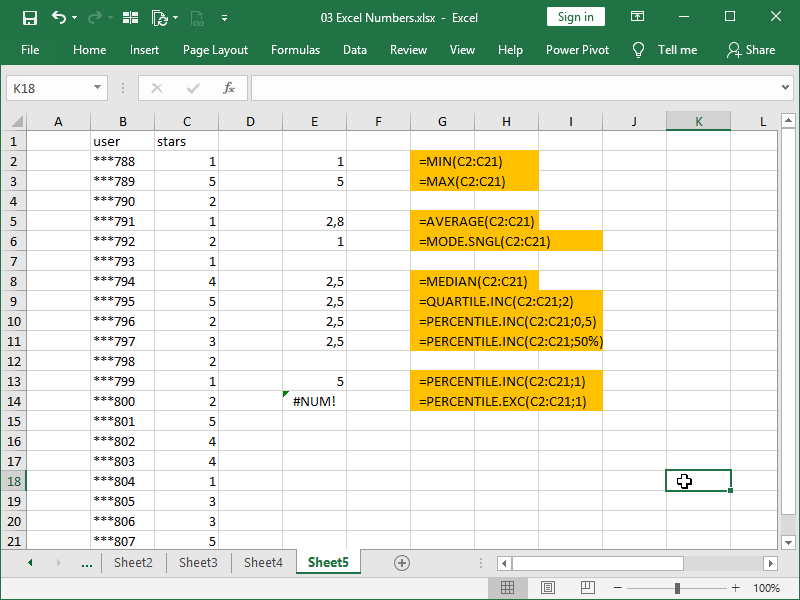Also note that:

• as PERCENTILE functions are basically general-purpose quantile functions, statements such as =PERCENTILE.INC(C:C;0,95674) are valid
• you can write in a % instead of a number between 0 an 1 in the PERCENTILE functions and still return the correct result
• as .EXC functions exclude both endpoints of the range (0,1), you can not return minimum and maximum with them.